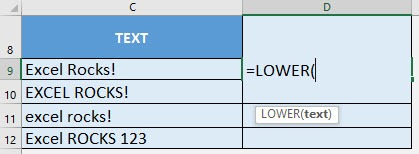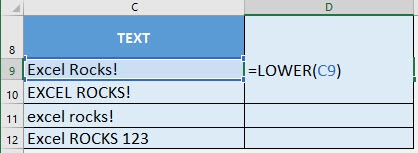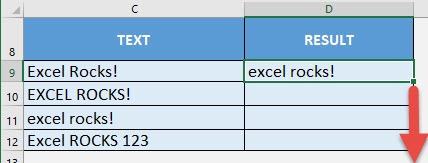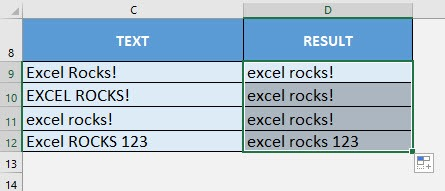What does it do?

Converts all characters in the text into lowercase

Formula breakdown:

=LOWER(text)

What it means:

=LOWER(text to be converted to lower case)

We can easily change text into lower case using the LOWER formula in Excel. It does not convert symbols and numbers though which we will see in the examples.

If you want to get rid of uneven capitalization throughout the text, then this formula is perfect for you!

I explain how you can do this below:STEP 1: We need to enter the LOWER function in a blank cell:

## =LOWER(STEP 2: The LOWER arguments:

## text

What is the text to be converted to lower case?

Select the cell containing the text that you want to convert:

## =LOWER(C9)Apply the same formula to the rest of the cells by dragging the lower right corner downwards.You can see that the values are all now in lower case!How to Use the LOWER Formula in Excel